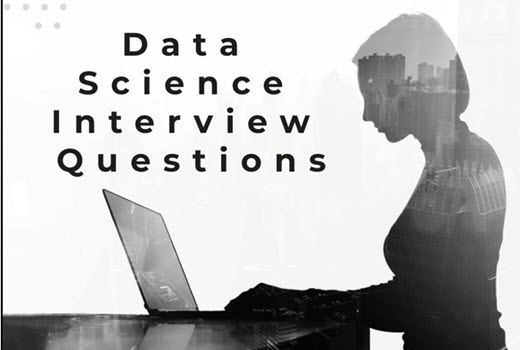#### Top 20 Data science Interview questions1. What is Data Science?

Data Science is a combination of algorithms, tools, and machine learning technique which helps you to find common hidden patterns from the given raw data.

2. What is logistic regression in Data Science?

Logistic Regression is also called as the logit model. It is a method to forecast the binary outcome from a linear combination of predictor variables.

3. Name three types of biases that can occur during sampling

In the sampling process, there are three types of biases, which are:

• Selection bias
• Under coverage bias
• Survivorship bias

4. Discuss Decision Tree algorithm

A decision tree is a popular supervised machine learning algorithm. It is mainly used for Regression and Classification. It allows breaks down a dataset into smaller subsets. The decision tree can able to handle both categorical and numerical data.

5. What is Prior probability and likelihood?

Prior probability is the proportion of the dependent variable in the data set while the likelihood is the probability of classifying a given observant in the presence of some other variable.6. Explain Recommender Systems?

It is a subclass of information filtering techniques. It helps you to predict the preferences or ratings which users likely to give to a product.

7. Name three disadvantages of using a linear model

Three disadvantages of the linear model are:

• The assumption of linearity of the errors.
• You can’t use this model for binary or count outcomes
• There are plenty of overfitting problems that it can’t solve

8. Why do you need to perform resampling?

Resampling is done in below-given cases:

• Estimating the accuracy of sample statistics by drawing randomly with replacement from a set of the data point or using as subsets of accessible data
• Substituting labels on data points when performing necessary tests
• Validating models by using random subsets

9. List out the libraries in Python used for Data Analysis and Scientific Computations.

• SciPy
• Pandas
• Matplotlib
• NumPy
• SciKit
• Seaborn

10. What is Power Analysis?

The power analysis is an integral part of the experimental design. It helps you to determine the sample size requires to find out the effect of a given size from a cause with a specific level of assurance. It also allows you to deploy a particular probability in a sample size constraint.

11. Explain Collaborative filtering

Collaborative filtering used to search for correct patterns by collaborating viewpoints, multiple data sources, and various agents.

12. What is bias?

Bias is an error introduced in your model because of the oversimplification of a machine learning algorithm.” It can lead to underfitting.

13. Discuss ‘Naive’ in a Naive Bayes algorithm?

The Naive Bayes Algorithm model is based on the Bayes Theorem. It describes the probability of an event. It is based on prior knowledge of conditions which might be related to that specific event.

14. What is a Linear Regression?

Linear regression is a statistical programming method where the score of a variable ‘A’ is predicted from the score of a second variable ‘B’. B is referred to as the predictor variable and A as the criterion variable.

15. State the difference between the expected value and mean value

They are not many differences, but both of these terms are used in different contexts. Mean value is generally referred to when you are discussing a probability distribution whereas expected value is referred to in the context of a random variable.

16. What the aim of conducting A/B Testing?

AB testing used to conduct random experiments with two variables, A and B. The goal of this testing method is to find out changes to a web page to maximize or increase the outcome of a strategy.

17. What is Ensemble Learning?

The ensemble is a method of combining a diverse set of learners together to improvise on the stability and predictive power of the model. Two types of Ensemble learning methods are:

Bagging

Bagging method helps you to implement similar learners on small sample populations. It helps you to make nearer predictions.

Boosting

Boosting is an iterative method which allows you to adjust the weight of an observation depends upon the last classification. Boosting decreases the bias error and helps you to build strong predictive models.

18. Explain Eigenvalue and Eigenvector

Eigenvectors are for understanding linear transformations. Data scientist need to calculate the eigenvectors for a covariance matrix or correlation. Eigenvalues are the directions along using specific linear transformation acts by compressing, flipping, or stretching.

19. Define the term cross-validation

Cross-validation is a validation technique for evaluating how the outcomes of statistical analysis will generalize for an Independent dataset. This method is used in backgrounds where the objective is forecast, and one needs to estimate how accurately a model will accomplish.

20. Explain the steps for a Data analytics project

The following are important steps involved in an analytics project: